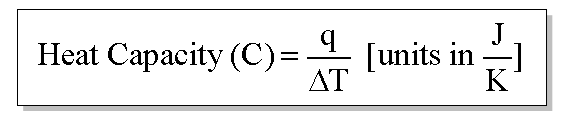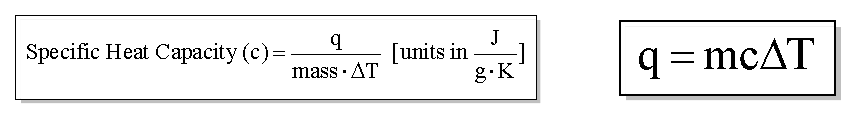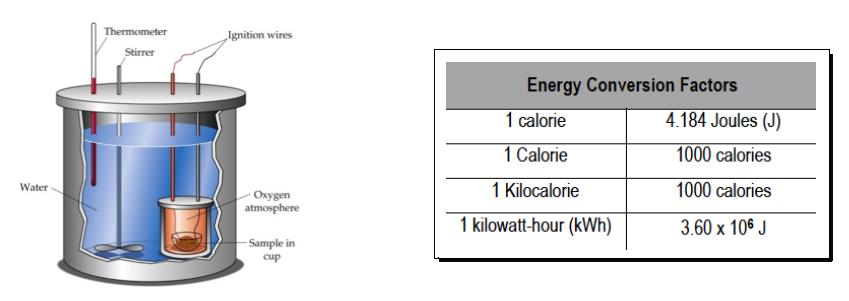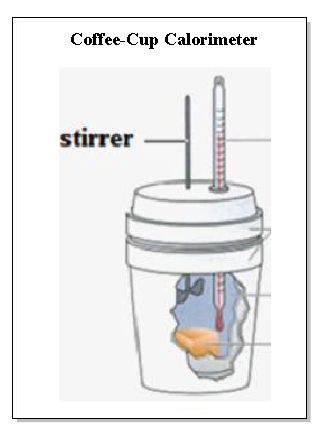Ch.6 - Thermochemistry WorksheetSee all chapters
 Ch.1 - Intro to General Chemistry 2hrs & 53mins 0% complete Worksheet Ch.2 - Atoms & Elements 2hrs & 40mins 0% complete Worksheet Ch.3 - Chemical Reactions 3hrs & 25mins 0% complete Worksheet BONUS: Lab Techniques and Procedures 1hr & 38mins 0% complete Worksheet BONUS: Mathematical Operations and Functions 47mins 0% complete Worksheet Ch.4 - Chemical Quantities & Aqueous Reactions 3hrs & 30mins 0% complete Worksheet Ch.5 - Gases 3hrs & 47mins 0% complete Worksheet Ch.6 - Thermochemistry 2hrs & 28mins 0% complete Worksheet Ch.7 - Quantum Mechanics 2hrs & 35mins 0% complete Worksheet Ch.8 - Periodic Properties of the Elements 1hr & 57mins 0% complete Worksheet Ch.9 - Bonding & Molecular Structure 2hrs & 5mins 0% complete Worksheet Ch.10 - Molecular Shapes & Valence Bond Theory 1hr & 31mins 0% complete Worksheet Ch.11 - Liquids, Solids & Intermolecular Forces 3hrs & 40mins 0% complete Worksheet Ch.12 - Solutions 2hrs & 17mins 0% complete Worksheet Ch.13 - Chemical Kinetics 2hrs & 22mins 0% complete Worksheet Ch.14 - Chemical Equilibrium 2hrs & 26mins 0% complete Worksheet Ch.15 - Acid and Base Equilibrium 4hrs & 42mins 0% complete Worksheet Ch.16 - Aqueous Equilibrium 3hrs & 48mins 0% complete Worksheet Ch. 17 - Chemical Thermodynamics 1hr & 44mins 0% complete Worksheet Ch.18 - Electrochemistry 2hrs & 58mins 0% complete Worksheet Ch.19 - Nuclear Chemistry 1hr & 33mins 0% complete Worksheet Ch.20 - Organic Chemistry 3hrs 0% complete Worksheet Ch.22 - Chemistry of the Nonmetals 2hrs & 1min 0% complete Worksheet Ch.23 - Transition Metals and Coordination Compounds 1hr & 54mins 0% complete Worksheet

# Calorimetry

See all sections
Sections
Internal Energy
Calorimetry
Thermochemical Equation
Hess's Law
Enthalpy of Formation
Units of Energy
Endothermic & Exothermic Reactions
Enthalpy

Both heat capacity (C) and specific heat capacity (c) deal with the amount of heat required to change a compound’s temperature by 1 Kelvin. Specific heat capacity (c) deals with changing 1 gram of a compound.

###### Constant Volume Calorimetry

By using calorimetry we can measure the thermal energy of a reaction (system) by measuring the change in heat of the surroundings.

Concept #1: Heat capacity vs. Specific Heat Capacity

Transcript

Welcome back guys! In this new video, we're going to take a look at calorimetry under a constant volume.
Now first we're going to say that every object has its own heat capacity. And just remember heat capacity uses a capital C, and this is just the amount of heat that's required to change an object's temperature by 1 Kelvin (K). Here we're just going to say heat capacity is simply heat/the change in temperature, so Final - Initial. And we're going to say the units are usually in Joules (J)/Kelvin or kiloJoules/Kelvin.
q J
C = ∆T [ in units of K ]

Sometimes your professor may even do it over Degrees Celsius, so just look to see what units were they want the answer to be in. That's what you always have to make sure so that you get the correct answer.
Now similar to heat capacity, there's another property. This property is known as Specific Heat Capacity, and notice that this one is lower case c, and this is the amount of heat that's required to change 1g of substance by 1K.
So here we're just going to introduce the concept of mass, and mass here would be in grams. So it's similar to heat capacity but more in depth, where talks about mass. Now what we're going to say here is if we know the specific heat capacity of a substance, we can rearrange this formula here so that we can solve for the amount of heat absorbed or released. So if we want to rearrange this equation up here, so C = q/m * change in temperature.
q
C = m * ∆T

Just multiply both sides by mass * change in temperature.
q
m * ∆T (C) = m * ∆T m * ∆T

And then you say that q = mcAT.
q = mc∆T
So just remember q = mcAT, just read the delta sign, the triangle as an A. So that's the easy way for you to remember the equation, q = mcAT. This is the equation that we use anytime they give us specific heat capacity, and we know that change in temperature, and they give us the mass of an object.
Now based on the concept we've just looked at let's take a look at this Question here. It says:
At constant volume, the heat of combustion of a particular compound is -4621.0 kJ/mol. We say when 2.319 grams of this compound (molar mass = 192.75 g/mol), remember it has a molar mass that I give to you, was burned in a bomb calorimeter, the temperature of the calorimeter (including its contents) rose by 3.138 oC. What is the heat capacity of the calorimeter in J/K?
First of all, this image that we have here is called a bomb calorimeter. It's a way of us actually figuring out how much energy does an object contain in it. The way it works is, we have our sample put inside to the small little bowl here, and that is placed inside of those water. This whole unit is referred to as a bomb. And what's going to happen is, we're going to actually use these rods here, and we're going to send an electrical current, and it's actually going to cause this sample to explode inside of the bomb calorimeter. So that's why it's called the bomb calorimeter because we actually cause the contents to explode. And when they explode, they're going to give off some heat. Now there's a temperature in the water, this temperature is actually going to record how much the water temperature increases by. In that way, we can figure out how much heat that that sample release and from that we can figure out how many calories it has, how much heat it started with, we could find out a lot of information. This is the way to do certain food sciences, to figure out the calories we have in a food. They use similar technique such as this.
Now we talked about Joules as being the standard type of energy for heat, but remember you could also have instead of Joules for q you could have kiloJoules, but you could also have calories, large calories, these are the calories that you see in food, and kilowatt-hours. So these are other units, energy conversion factors that we can use in place of Joules for q. Now we're not going to work on converting from Joules to calories or kilowatt-hours, I just want to give you guys these tables so that you can see all the different types of energy conversions that you should know for lecture.
Now let's take a look at this question, we want to calculate heat capacity. Remember this is capital C, and so heat capacity = q/change in temperature. And what we need to realize here is, we need to isolate Joules, we need to find Joules for q, and we need the temperature to be in Kelvin. We already have half of it right off the bat, the easy part. The easy part here is I told you that the temperature rose by this much.Example #1: Bomb Calorimeter

Transcript

That right there represents our change in temperature, but the thing is we just need to change those oC into K. And how do we do that? Remember the goal from oC to K, you just add 273.15 to it, when we do that we get 276.288 K.
3.138 oC + 273.15 = 276.288 K
Now what we have to do is we have to isolate q, we have to find Joules. Now the closest place we're going to find Joules are right here in kiloJoules. So we need to find a way of isolating those kiloJoules there. Now the only way I can isolate those kiloJoules is if I find a way to cancel out these moles that we have. And the way I'm going to cancel out those moles is if I convert these grams that I gave to you into moles and multiply times this whole thing. So what we're going to do is we're going to start out with 2.319 g, now we can change this into moles because I gave you the molecular weight, the molar mass of the object. So we're going to say for every 1 mole of this substance it's 192.75 g, grams cancel out and we've just isolated our moles.
1 mol
2.319 g x 192.75 g

I'm going to take myself out of the image so that we'll have more room to work with guys. So we have moles now, now I can just multiply times the heat of combustion, so this would be -4621.0 kJ on top, 1 mol on the bottom. Moles cancel out and now I have kiloJoules isolated.
1 mol -4621.0 kJ
2.319 g x 192.75 g x 1 mol

But remember I don't want kiloJoules, I want Joules. So one more step, kiloJoules go on the bottom, Joules on the top. Kilo is a metric prefix, so for every 1 kilo it's 103. When we work all of that out that gives me -55 600 J, so that's my q. So I'm going to plug it up here. So divide that by the temperature we just isolated and you'll get -201 J/K.
1 mol -4621.0 kJ 10J
2.319 g x 192.75 g x 1 mol x 1 kJ

= - 55 600 J

So I'm going to plug it up here. So divide that by the temperature we just isolated and you'll get -201 J/K.
q
C = ∆T
= - 55 600 J
276.288 kJ
= - 201 J/K

Now this question seems like a lot of information into this, but the thing is just focus on what they're asking us to find, they wanted us to find heat capacity. Heat capacity is just simply q/change in temperature, we knew change in temperature immediately so we just convert it that to Kelvin and then you're now to work on isolating the one variable you needed, you needed kJ, you need to isolate kJ. We isolated that by changing the grams I gave you into moles and then multiplying times the heat of combustion. Now when they say heat of combustion, remember heat means q, but more importantly here it means delta H. They could have said that enthalpy of combustion, also that be the same exact thing. Okay so just remember heat and enthalpy is the same thing.

A bomb calorimeter measures the amount of calories within a substance through combustion. In other words, we “blow it up” and measure the amount of heat it releases.###### Constant Volume-Calorimetry Calculations

If you are given energy or heat, with specific heat capacity and mass then you will most likely use q = mcΔT.

Example #2: In an experiment a 9.87 carat (1 carat = 0.200g) diamond is heated to 72.25oC and immersed in 22.08 g of water in a calorimeter. If the initial temperature of the water was 31.0oC what is the final temperature of the water? (cdiamond = 0.519) (cwater = 4.184 ).

Practice: A sample of copper absorbs 35.3 kJ of heat, which increases the temperature by 25 degrees Celsius, determine the mass (in kg) of the copper sample if the specific heat capacity of copper is 0.385 J/g *C.

When dealing with heat it is important to remember that if one compound is gaining heat that means another compound is losing heat.

Practice: 50.00 g of heated metal ore is placed into an insulated beaker containing 822.5 g of water. Once the metal heats up the final temperature of the water is 32.08 degrees Celsius. If the metal gains 14.55 kJ of energy, what is the initial temperature of the water?

###### Constant Pressure Calorimetry

The enthalpy or heat of a reaction can be calculated through the use of a coffee cup calorimeter.

Concept #2: Coffee Cup Calorimeter

Transcript

Welcome back, guys! In this new video, we're going to take a look at chemical reactions when the calorimetry is done under constant pressure. What you’re going to see here is this image right here. We're going to say that this is called a coffee calorimeter. We're going to say it’s used basically to help us find the enthalpy of a reaction. Remember, enthalpy just means delta H. The coffee cup calorimeter has certain parts to it. Here we have the stirrer, which just helps us to mix the water that surrounds our sample. Here we’re going to have our thermometer, which measures the temperature change involved when the sample undergoes its chemical reaction. The sample was going to be releasing heat. The water is going to be absorbing that heat. The thermometer is just to calculate the change in temperature that the water undergoes. Here we’re going to have the two styrofoam cups that are placed together. They work as insulation. That way, no heat is lost to the outside environment. All the temperature or energy changes that occur are going to happen between the water and the sample. Here we're going to have our water. Our water acts as the surroundings. Here we're going to have our sample, which is going to undergo the chemical reaction. This represents our system.
Remember, under constant pressure it's possible for us to calculate the enthalpy of the reaction through the use of this coffee cup calorimeter.Concept #3: Calculating the Heat of the Solution

Transcript

Now let's take a look at it this example question. Here it says you place 50 ml of 0.100 molar NaOH into a coffee cup calorimeter at 50 degrees Celsius and carefully add 75 mls of 0.100 molar hydrochloric acid also at 50 degrees Celsius. After stirring, the final temperature of the solution is 76.12 degrees Celsius. Heat capacity and the density of water are also given.
From this, we have to calculate two parts. For part A, we have to figure out what is the heat or Q of the solution in joules. Since we're going to need room to do both of these questions, I'm going to take myself out of the image guys, so we have more room to work with.
What we should realize is that we need to find the heat of the solution. The problem is it's hard to do it directly. But we can find the Q of the water. If we know Q of water, we can then say Q of water equals negative Q of the solution. Remember, in this process, the sample will undergo a chemical reaction and release heat that's why it’s negative. The water will absorb that heat that's why it's positive. We can find the Q of water because we can say Q of water equals mCΔT. We already know the specific heat water. I gave it to you. I said it’s 4.184 joules over degrees times Celsius. Our temperature change, our final temperature is 76.12 degrees Celsius. Our initial for both samples when we mix them together were 50 degrees Celsius.
The harder part is just figuring out what the mass of the water is. But we can figure this out because when we say 50 ml and 75 ml, those two things actually represent the volume of water. What's really going on here that we have that many milliliters of water and dissolved within it are those moles of NaOH and HCl. We're going to take those 50 mls and that 75 ml and add them together to give me 125 mls of water. Since I know the density of water here, I can use that to find the grams of water. Multiply this times the density. Mls cancel out and I have 125 grams of water, which I can just plug into here.
When I multiply everything out, that gives me 13,660.8 joules as the Q of water. We can just plug it into here so we’re going to have 13,660.8 joules equals negative Q of solution. But I want Q of solution, not negative Q of solution. So then all you have to do is divide both sides by -1. Now, Q of solution equals negative 13,660.8 joules as your final answer. Here we’re not concerning ourselves with number of significant figures because here we’re just trying to figure out what the answer is. If you will get this type of question on your exam, your professor would specify do they want sig figs or not.

The heat of the solution can be determined by first determining the heat of water

Concept #4: Calculating the enthalpy of the reaction

Transcript

For B, we have to calculate the enthalpy, delta H, in joules per mole for the formation of water. Here we have to figure out the enthalpy of water. We're going to say delta H of reaction equals Q of solution divided by the moles of whatever they're asking us to find. Here, they’re asking for the formation water so it’s moles of water. We already have half of this because we just figured out what the Q of solution was, so we’ll just plug it in. Now what we have to do is we have to figure out how many moles of water we have.
What we should realize here is I give you information on NaOH and HCl. Remember, the word of when it’s in between two numbers means multiply. Remember, when I say big M that means molarity. Molarity equals moles over liters. When I say 0.100 molar NaOH, that really means 0.100 moles of NaOH over 1 liter. We always assume it's always moles over liters.
If you guys don’t remember this too well, go back to a few videos past where we talked about molarity and molarity in stoichiometry because to do this question, it's essential that you remember those steps from earlier. We’re going to work it out down here. Since I gave you information on two reactants and I'm asking you how much product do you have in moles, this is really a limiting reactant type of question. We're going to have to first do it with the molarity of NaOH, see how many moles of water that gives us then do the same thing with HCl. Since this is a limiting reactant type of question, the one that gives us a smaller amount of moles of water, those are those moles we’re going to use. We have 50 ml of 0.100 molar NaOH. I need to change my ml into liters. 1 ml on the bottom, 10 to the negative 3 liters on the top. Mls cancel out and I finally isolate liters. Now that I have liters, I can multiply it times the molarity to find the moles of NaOH. We need the moles of water though. We need to go from these moles I've given that we just found to our moles of unknown. Remember when we do that jump, we do a mole to mole comparison. We look at the coefficients in the balanced equation. According to our balanced equation, for every one mole of NaOH, we have one mole of water. NaOH on the bottom and mole of H2O what we’re looking for in the top. Since this is in 10 to the negative 3, put it in brackets. When we work it out, we get 0.005 moles of water.
Now we have to do the same thing with HCl. We have 75 ml of 0.100 molar HCl. Same thing, we change the mls into liters first then we multiply it times the molarity. For every one mole of HCl, we have one mole of H2O. Liters cancel out, moles cancel out. We have 0.0075 moles of water. Remember, since this is the limiting reactant type of question, we're going to go with the smaller moles of water. Those are the moles we're going to plug down here to find our enthalpy. When we plug that in, we get back negative 2.73 times 10 to the 6 joules per mole.
This question had a lot of parts to it. It became even harder because we had to incorporate concepts that we learned a few videos back. Just because we passed molarity and stoichiometry involved with them doesn't mean we don't have to see it again this semester. Those types of ideas always resurface at some point. We had to use them in order to solve this question. Remember, if we’re at constant pressure, that's when we use a coffee cup calorimeter. Its whole purpose is to help us find the enthalpy of a reaction. Remember, if your enthalpy, I may not have said that but if your enthalpy is positive, that means you’re absorbing energy or absorbing heat so you're endothermic. If you're releasing heat or releasing energy by the system, then it's exo. It’s negative delta H. Just remember the concepts that we covered here and the approaches we take. When you see a question like this, you'd be better prepared to do it.